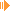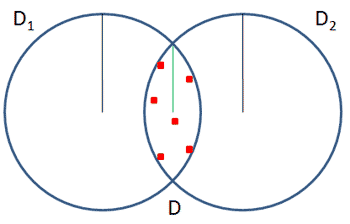Computing the smallest enclosing disk in 2DDownload Applet with Source (26 kb)

Instructions:
* By left-clicking in the blue area, you can set new points.
* By right-clicking points you can remove them.
* Note that they are also draggable.
* If you don't want to set many points manually, you can create a specified number of random points automatically.
* The Clear-Button removes all points.
* Finally pressing the Go-Button calculates and draws the minimal enclosing circle.

Notes:

The applet you see above is my own implementation of Welzl's algorithm which computes the minimal enclosing circle. I came around this algorithm while learning for my oral exam in Computer Graphics and I thought why not trying to visualize it.
This algorithm was presented by Welzl in 1991  and runs (in contrast to most other algorithms for solving this prolbem) in linear time!So what it is all about? Let's say we have a set P of n points in the plane, we want to calculate the closed disk of smallest radius D(P) which contains all points in P. Look at the picture left: we have a number of red points; the blue circle is the smallest one which contains all red points. So how to find this smallest circle (which means how to find its center and its radius) - that's the question!

First of all, it's easy to prove that such a smallest enclosing disk (sed) is unique. Assume we have two smallest disks D1and D2. That means that each disk contains the points in P, so also the intersection D с D1u D2 must contain them. But a circle around D has a smaller radius than the disks D1and D2, so our assumption of two different smallest disks fails and we proved the uniqueness. The figure below should make it clear:The algorithm works now step by step: assume we know already a sed (smalled enclosing disk) D for n-1 points p1,...,pn-1. Now there are two cases for the nth point.
1) pn lies inside D. So nothing changes - the sed D for p1,...,pn-1 is the same for p1,...,pn!
2) pn lies NOT inside D. So we have to compute a new sed. But we know (that's a fact) that pn must lie on the boundary of D, call it BD! So we have to calculate a sed D' for p1,...,pn-1 with pn on the boundary of D'.

In fact, that's the main idea. This property along with the three following claims allows us to calculate such a smallest enclosing disk in an iterative way:
Let P again be a set of n points, P is not empty and p is a point in P. R is also a set of points, in fact these are the points on the boundary of the disk. Then the Lemma says:
(i) If there exists a disk containing P with R on its boundary, then D(P,R) is well defined = unique.
(ii) If p lies not in D(P - {p},R), then p lies on the boundary of D(P,R), provided it exists, meaning:
D(P,R) = D(P - {p}, R u {p}).
(iii) If D(P,R) exists, there is a set S of most max{0, 3 - |R|} points in P such that D(P,R) = D(S,R). That means that P is determined by at most 3 points in P which lie on the boundary of D(P).

With this information, one can implement this algorithm in a recursive way. Of course, as in all recursions, there are terminal or end cases in which you can calculate the solution directly. Here it is if we have 3 points only: a minimal circle in this case has the 3 points on the boundary and it's definitely determined (if the three points lie not on a common line!). In my implementation I also considered the cases with only 1 and 2 points. In fact I found it quite difficult to get the algorithm working - curius was that when I used arrays to hold the points the recursion works, but when I used vectors very strange things happened... Nevertheless, now it works and I hope you found my notes along the applet and its sources useful!

Pseudocode:

 /*  * Calculates the sed of a set of Points. Call initially with R = empty set.  * P is the set of points in the plane. R is the set of points lying on the boundary of the current circle.  */ function sed(P,R) {     if (P is empty or |R| = 3) then          D := calcDiskDirectly(R)     else         choose a p from P randomly;         D := sed(P - {p}, R);         if (p lies NOT inside D) then             D := sed(P - {p}, R u {p});     return D; }

References:

 Smallest enclosing disks (balls and ellipsoids), Emo Welzl, 1991

Sunshine, May 2008

This site is part of Sunshine's Homepage